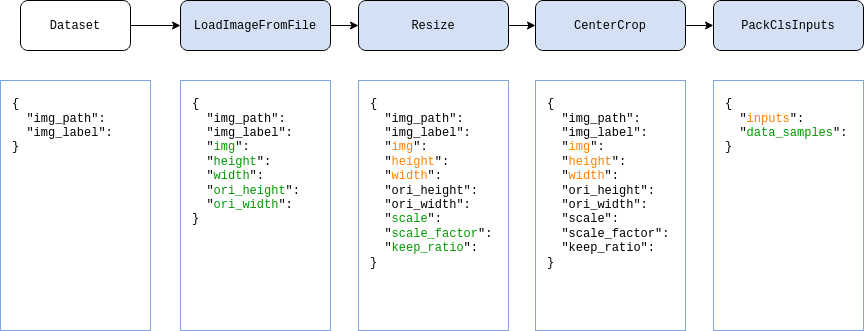Shortcuts

# 数据变换 (Data Transform)¶

## 使用数据变换类¶

MMEngine 中仅约定了数据变换类的规范，常用的数据变换类实现及基类都在 MMCV 中，因此在本篇教程需要提前安装好 MMCV，参见 MMCV 安装教程

>>> import numpy as np
>>> from mmcv.transforms import Resize
>>>
>>> transform = Resize(scale=(224, 224))
>>> data_dict = {'img': np.random.rand(256, 256, 3)}
>>> data_dict = transform(data_dict)
>>> print(data_dict['img'].shape)
(224, 224, 3)


## 在配置文件中使用¶

1. 数据加载，通常使用 LoadImageFromFile

2. 标签加载，通常使用 LoadAnnotations

3. 数据处理及增强，例如 RandomResize

4. 数据格式化，根据任务不同，在各个仓库使用自己的变换操作，通常名为 PackXXXInputs，其中 XXX 是任务的名称，如分类任务中的 PackClsInputstest_dataloader = dict(
batch_size=32,
dataset=dict(
type='ImageNet',
data_root='data/imagenet',
pipeline = [
dict(type='Resize', size=256, keep_ratio=True),
dict(type='CenterCrop', crop_size=224),
dict(type='PackClsInputs'),
]
)
)


## 常用的数据变换类¶

### 数据加载¶

LoadImageFromFile

LoadAnnotations

### 数据预处理及增强¶

Pad

CenterCrop

Normalize

Resize

RandomResize

RandomChoiceResize

RandomGrayscale

RandomFlip

### 数据格式化¶

ToTensor

ImageToTensor

## 自定义数据变换类¶

import random
import mmcv
from mmcv.transforms import BaseTransform, TRANSFORMS

@TRANSFORMS.register_module()
class MyFlip(BaseTransform):
def __init__(self, direction: str):
super().__init__()
self.direction = direction

def transform(self, results: dict) -> dict:
img = results['img']
results['img'] = mmcv.imflip(img, direction=self.direction)
return results


import numpy as np

transform = MyFlip(direction='horizontal')
data_dict = {'img': np.random.rand(224, 224, 3)}
data_dict = transform(data_dict)
processed_img = data_dict['img']


pipeline = [
...
dict(type='MyFlip', direction='horizontal'),
...
]


© Copyright 2022, mmengine contributors. Revision f356b3c2.

Built with Sphinx using a theme provided by Read the Docs.
Versions
latest
stable
v0.7.0
v0.6.0
v0.5.0
v0.4.0
v0.3.0
v0.2.0